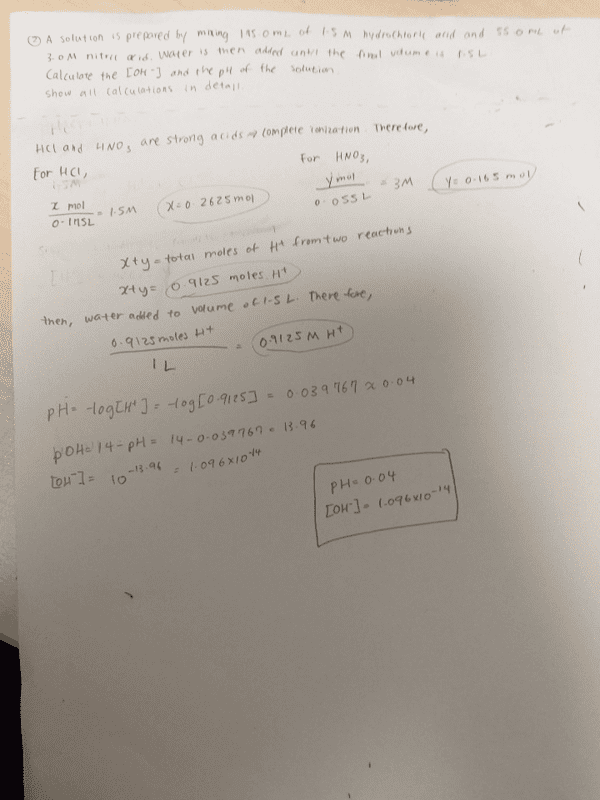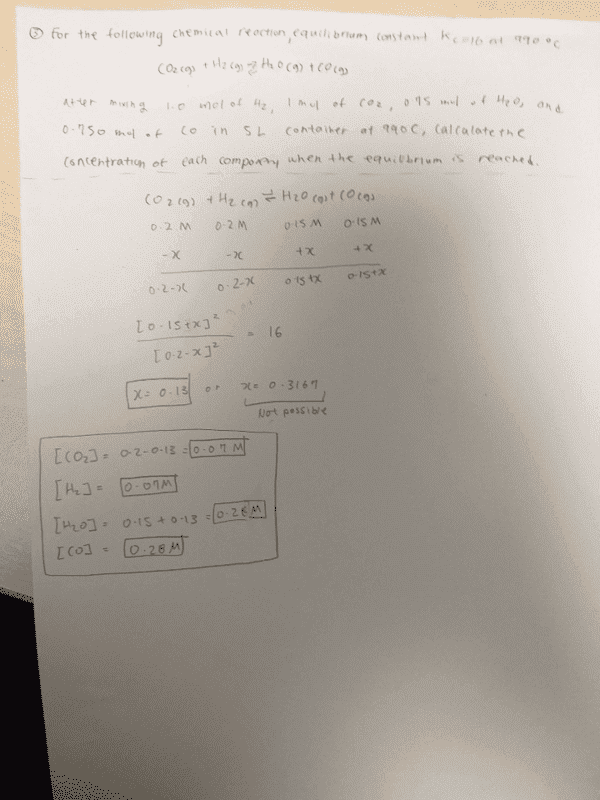# Can anyone check my answers for these equilibrium problems?

• Chemistry
• Sunwoo Bae

#### Sunwoo Bae

Homework Statement
2.A solution is prepared by mixing 175.0mL of a 1.5 M hydrochloric acid and 55.0 mL of a 3,0 M nitric acid. Water is then added until the final volume is 1.50L. Calculate the [OH-] and the pH of the final solution.
Show all calculations in detail.

3. For the following chemical reaction equilibrium constant (Kc) is 16 at 990C.
CO2(g) + H2(g) --> H2O(g) + CO(g)

After the mixing of 1.00 mol of H2, 1.00 mol of CO2, 0.750 mol of H2O, and 0.750 mol of CO in 5.00L container at 990 C, calculate the concentrations of each component when the equilibrium is reached.
Show all calculations in detail.
Relevant Equations
ICE setup

pH + pOH = 14
pH = -log(H+)
pOH = -log(OH-)
The following are my works for the problems:
I put squares on my final answers!Thank you!

I definitely agree with your answer to (3); I also agree with your method for (2), though I suspect there might have been a numerical error. Specifically, shouldn't it be ##x + y = 0.4275 \text{ mol}##? It could be that you entered ##y = 0.65 \text{ mol}## into your calculator instead of ##y = 0.165 \text{ mol}##

•Sunwoo Bae
Also, when calculating the concentration, you should divide by 1.5 L, not 1 L.

•etotheipi